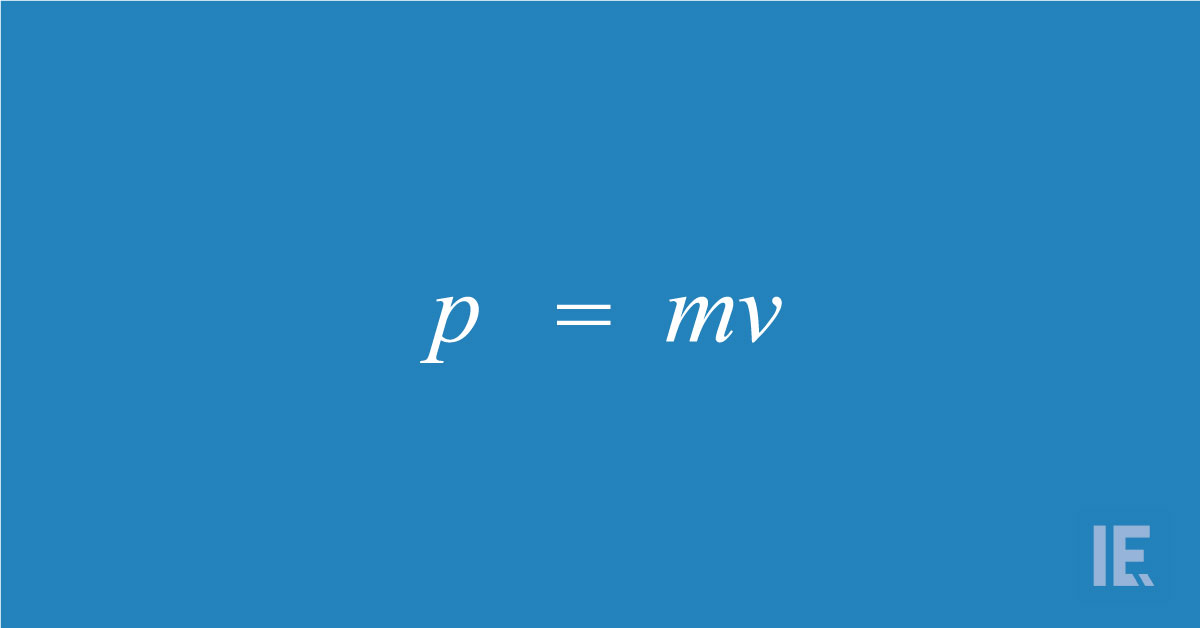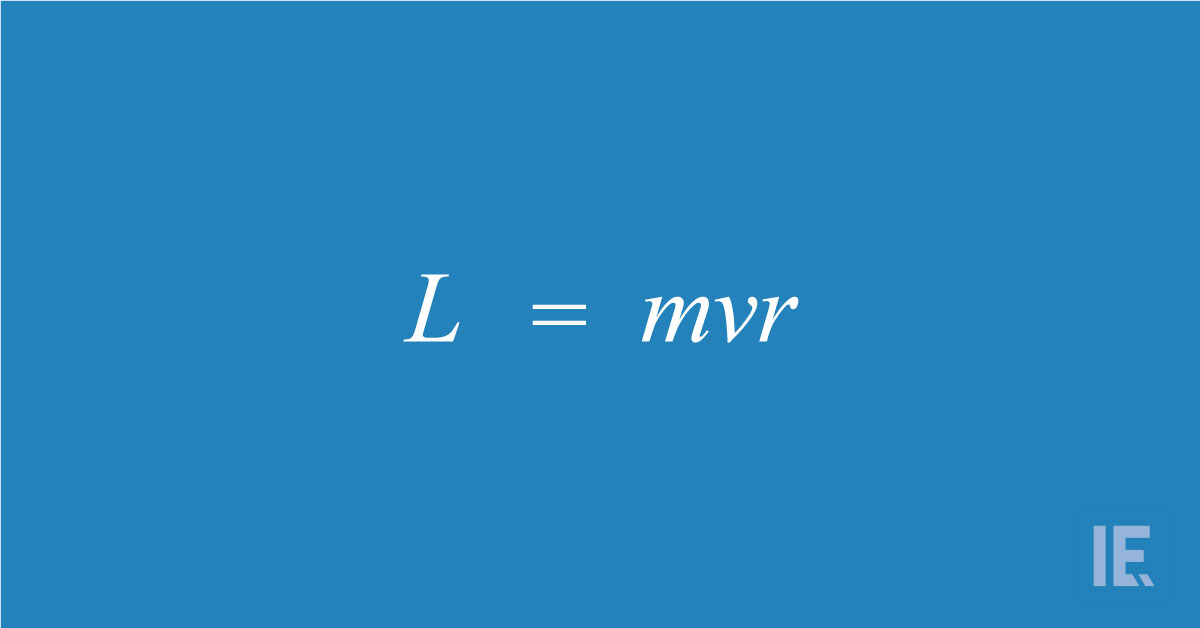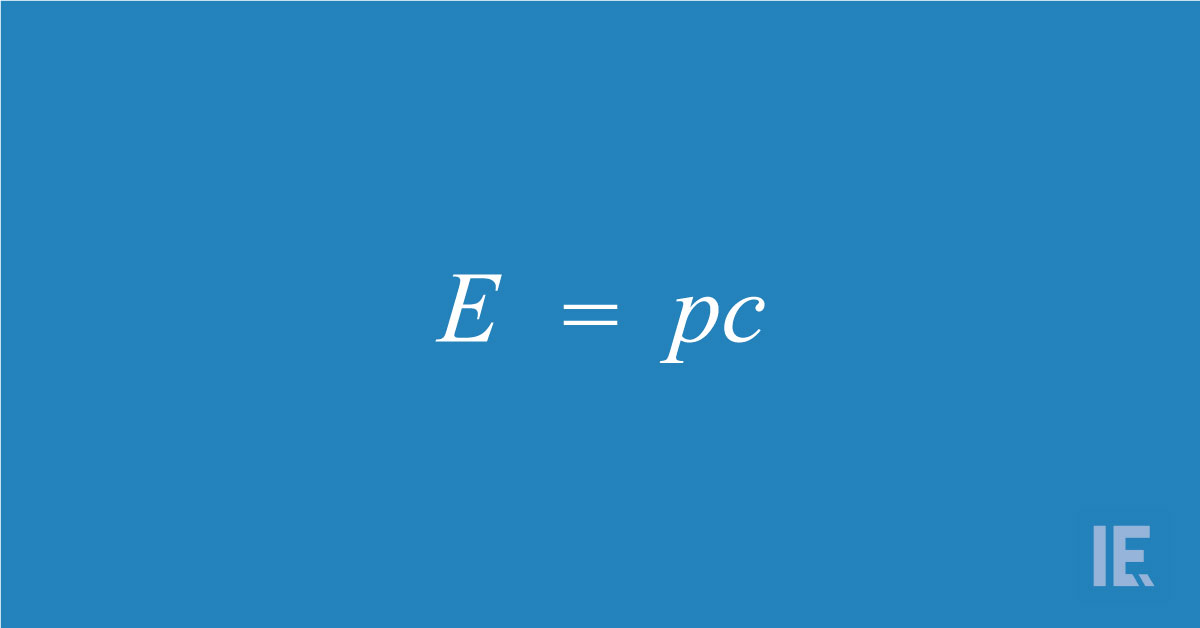# Momentum in Physics: Newton’s Laws of Motion, Collisions, and MoreMomentum is a term used in physics to describe the quantity of motion of a moving body, measured as a product of its mass and velocity.

RELATED: 9+ OF THE BEST YOUTUBE CHANNELS FOR PHYSICS LOVERS

## What is momentum?

Moving objects have momentum. This is the tendency of the object to keep moving in the same direction unless acted on by an outside force. Momentum is the product of the mass of a body and its velocity. Since it has both magnitude and direction, momentum is a vector quantity.

That means that momentum has both magnitude and direction. The equation for Momentum is given below.In this equation:

p is momentum
m is mass
v is the velocity

However, momentum does not just depend on the object’s mass and speed. Because velocity is speed in a particular direction, the momentum of an object also depends on the direction of travel. This means that the momentum of an object can change if the object speeds up or slows down; or if it changes direction.

This means that we can think of momentum in two ways, based on the movement of the object.

## 1.      Linear Momentum

Linear momentum is the momentum of a body moving in a straight line. When we write the general equation of momentum (above), we use the equation for linear momentum.

## 2.      Angular Momentum

Angular momentum is the momentum of a body moving at an angle. In this case, the mass of the body is the same, however, we replace velocity with angular velocity.

This creates a change in the equation. Angular momentum is also created when an object spins on its axis. For example, a spinning top possesses angular momentum when it spins on its own axis, even it doesn’t move from the spot.

The equation for angular momentum is as follows:In this equation:

L is angular momentum
m is mass
v is velocity

## The curious case of light: momentum without mass

We have already discussed that momentum is dependant on mass and velocity. If anyone of these two values is zero, then the momentum is also zero.

However, there is an exception to this – light. Light has no mass, but it does carry momentum. We do not see things falling over when light shines on them, because the momentum of light is very small, but it is measurable.In fact, for photons (the smallest bits of light), the energy E and momentum p are related by the equation:Where:

E is energy
P is momentum
c is the speed of light

The momentum of light is so small that we don't notice it in everyday life. But it is large enough to be measurable, and can even be put to use. For example, laser cooling machines use the momentum of light from a to slow down atoms in a sample, thereby cooling it. In optical traps, the momentum of the light is used to trap and manipulate small objects.

## What is the moment of inertia? Is it connected to momentum?

So, how does the moment of inertia, a rotating body's resistance to angular momentum, relate to momentum? Is it the same as momentum, or is it something else entirely?

Inertia is an object’s resistance to a change in motion. According to Newton's first Law, a body will remain at rest or in uniform motion in a straight line unless acted upon by an external force. Inertia is a scalar quantity, which means it has  only magnitude, not direction.

The moment of inertia expresses a body's is an object’s tendency to resist angular acceleration, which is the sum of the products of the mass of each particle in the body with the square of its distance from the axis of rotation.

## Momentum in Newton’s Laws of Motion

Momentum plays an important part in forming Newton’s Second and Third Laws of Motion.

Newton’s second law states that the acceleration of an object, as produced by a net force, is directly proportional to the magnitude of the net force, in the same direction as the net force, and inversely proportional to the mass of the object.

Another way of saying this is that the rate of change of momentum in an object is directly proportional to the force applied, and the change in momentum will be in the direction of the applied force.

Newton’s third law states that for a force applied by an object A on object B, object B exerts back an equal force in magnitude, but opposite in direction. This idea was used by Newton to derive the law of conservation of momentum. It is often stated as: For every action, there is an equal and opposite reaction.

The law of conservation of momentum states that if two objects collide with each other, the combined momentum of the objects before collision will be equal to the combined momentum of the two objects after the collision.

In other words, the momentum of an isolated system will always remain the same. The combined momentum stays the same because the momentum lost by object A will be gained by object B.

## Different types of collision

You might be surprised when we said that the momentum lost by an object A will be gained by object B. We don’t see that happen in real life!

That is because there are two types of collisions.

Elastic collision: Elastic collision is the type of collision where the two objects collide and there is a transfer of energy from one object to another, but no net loss of kinetic energy. For example, two similar balls are traveling toward each other with equal speed. They collide, bouncing off each other with no loss in speed. This collision is an ideal case because no energy has been lost.

An perfectly elastic collision is not possible in everyday life, as there are other forces at play which cause energy to be lost through friction, heat, etc. There are some examples of collisions in mechanics where the energy lost is very small, and can be considered elastic, even though they are not perfectly elastic.

Inelastic collision: In an inelastic collision, part of the kinetic energy is changed to some other form of energy, such as like heat or sound. Instead of bouncing back, the object tends to stick together. Momentum is conserved in inelastic collisions.

The collisions that we see in our day-to-day lives fall between elastic and inelastic collisions.

## The importance of momentum in science

Momentum is an important consideration in physics because it describes the relationship between speed, mass and direction.

Momentum describes the force needed to stop objects and to keep them in motion. For example, it explains that you need to exert more force to stop an object with greater momentum, when compared to an object having lower momentum. Hence, momentum is an important factor to consider when we design systems to stop a moving object.

A seemingly small object can exert a large amount of force if it has enough momentum. One of the best examples of this phenomenon is a bullet. Momentum can also be used to predict the resulting direction and speed of motion of objects after they collide.